# An Intro to Solving Linear Equations: Solving some Basic Linear Equations

##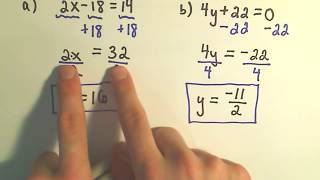By patrickJMT

An Intro to Solving Linear Equations: Solving some Basic Linear Equations# Solving matrix equations

##By Khan Academy

After he represented a system of equations with a single matrix equation, Sal solves that matrix equation using the inverse of the coefficient matrix.# Radical equations

##By Khan Academy

Sal explains what square-root equations are, and shows an example of solving such an equation and checking for extraneous solutions.# Graphing Quadratic Equations

##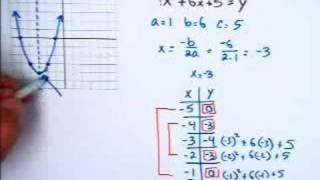By Marc Whitaker

Instructor uses a white board to model graphing quadratic equations. Examples show using quadratic equations in standard form to determine the line of symmetry, create a table of values, and graph the quadratic equation by using these values.# Graphing systems of equations | Systems of equations | 8th grade | Khan Academy

##By Khan Academy

This video describes graphing systems of equations.# Writing systems of equations as matrix equations

##By Khan Academy

Sal shows how a system of two linear equations can be represented with the equation A*x=b where A is the coefficient matrix, x is the variable vector, and b is the constant vector.# Absolute value equations 1 | Linear equations | Algebra I | Khan Academy

##By Khan Academy

Absolute Value Equations 1# Solving Systems of Equations using Substitution

##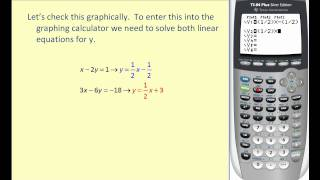By Mathispower4u

Solving Systems of Equations using Substitution# Solutions to quadratic equations

##By Khan Academy

Discriminant of Quadratic Equations# Graphing Systems of Equations Video: Graphing Systems of Equations

##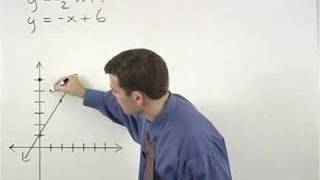By yourteachermathhelp

This lesson explains how to solve a system of two equations by graphing. The instructor begins by graphing the line for each equation. Then he demonstrates how to find the point of intersection which is the solution for the system.# Creating and Solving Equations

##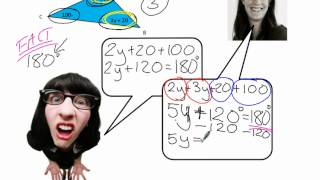By KSAMathematics

This video addresses common mistakes and misconceptions when creating and solving equations. The examples focus specifically on creating equations from geometric shapes.# Extraneous solutions to radical equations

##By Khan Academy

Sal explains what square-root equations are, and shows an example of solving such an equation and checking for extraneous solutions.# Equations with variables

##By MathPlanetVideos

Solve the following equation 8⋅x−x=21# Fundamentals in solving Equations in one or more steps

##By MathPlanetVideos

Solve the equation# Linear equations in the coordinate plane

##By MathPlanetVideos

Find the values of x and draw this equation in a graph# Solve systems of equations by graphing

##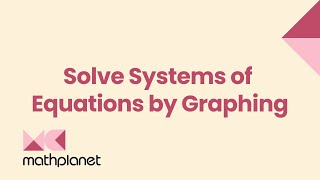By MathPlanetVideos

Find the solution of two equations by graphing# Solving Systems of Equations using Substitution

##By Mathispower4u

Solving Systems of Equations using Substitution# Writing Equations of Exponential Functions

##By drmikedcook

Writing Equations of Exponential Functions# Writing Equations of Exponential Functions

##By drmikedcook

Writing Equations of Exponential Functions# Equations of conic sections

##By MathPlanetVideos

Write the given equation on the standard equation form for ellipses